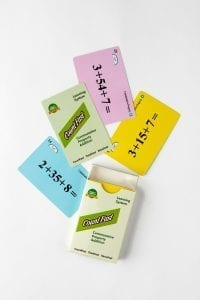# Commutative Property Addition Lesson Plan

####OVERVIEW & PURPOSE

Week 7 of the 2nd Grade CountFast program reviews using the Commutative Property of Addition to quickly solve equations with more than two numbers. This module includes equations to solve each day, with an emphasis on improving fluency and speed with basic facts. Spend 15 minutes each day on one of the activities listed in this module.  Card decks should go home with students each day for additional practice with a parent at home. Each week, a new deck is introduced and the previous deck is for the student to keep at home for continued practice.

#### EDUCATION STANDARDS

1.  NCTM Standard: develop fluency with basic number combinations for addition and subtraction
2.  NCTM Standard: understand the effects of adding and subtracting whole numbers
3.  CCSS.MATH.CONTENT.1.OA.C.6  Add and subtract within 20, demonstrating fluency for addition and subtraction within 10.

#### OBJECTIVES

Develop fluency in using the Commutative Property concept to quickly mentally calculate single-digit addition and subtraction equations.

#### MATERIALS NEEDED

1.  One CountFast Commutative Property card deck for each student.  This deck is for school and home use.  Discuss routine and expectations for taking home the deck and returning it to school each day.
2.  One writing utensil per student (optional, if you desire students to practice writing the equations)

Commutative Property Days 1 through 3

Teacher Model/Direct:  Use the yellow cards from the deck.  These have addition equations on them.  Hold up one equation at a time for the class to read and answer together. (If you are holding the card, 1 + 5 + 9 =, the children should say “One plus five equals 6, and nine more makes 15.”)  Remind students of the Commutative Property of Addition, and how this allows them to add up the addends in any order to get the same sum.  Look especially for way to make a group of 10 to make calculation easier.  The same card from above can be solved more quickly by saying, “One and nine make 10, and ten plus five makes 15.”  Practice together using 10 yellow cards each day.  Then repeat the activity, telling students you will be going through the cards faster this time, and you only want them to say the answer (without reading aloud the problem).  Repeat this for 2-3 rounds as a whole group.

Student Activity:  Partner up students and give each pair one deck to work with.  Ask them to take out ten yellow cards.  Students should take turns being the ‘card turner’ and the ‘solver’.  Have students practice positively encouraging one another, even when mistakes are made.  At the end of the Day 1 exercises, be sure to give each child a pack of the cards to take home for practice, along with the Home Connection letter for this week.

Home Activity: Students will take home the deck and the “CountFast Home Connection” letter.  Students will practice the addition equations on the yellow cards with parent(s).  Parents will time the child’s progress each round of play, using the YELLOW cards.

Commutative Property Days 4 and 5

Teacher Model/Direct:  Use the BLUE cards from the deck.  These have subtraction equations on them.  Hold up one equation at a time for the class to read and answer together. For cards with three digits in the equation, explain that the most important part of the problem is remembering that the beginning (1st) number is what you have to start with.  The other digits can be subtracted from that number in any order.  For example: 20 – 1 – 9 = 10.  This can be solved in left-to-right order by subtracting 1 from 20 to get 19, and then subtracting 9 from the remaining 19 to get a final difference of 10.  However, another strategy is to first add the 1 and 9 together to make 10, and then subtracting that total of 10 from the original number of 20.  Additionally, this can be solved by first subtracting the 9 from the 20 to get 11, and then subtracting the 1 to get a final difference of 10. Discuss ways that using the concept of Commutative Property of Addition can be use to solve multi-step subtraction problems (especially by looking for ways to group numbers to make 10).  Practice together using the BLUE cards.  Then repeat the activity, telling students you will be going through the cards faster this time, and you only want them to say the answer (without reading aloud the problem).  Repeat this for 2-3 rounds as a whole group.

Student Activity:  Partner up students and ask them to take out their BLUE cards.  Students should take turns being the ‘card turner’ and the ‘solver’.  Have students practice verbalizing the subtraction process for each card, and positively encouraging one another, even when mistakes are made.  At the end of this activity, remind students to take the pack home for practice.

Home Activity: Students will take home the deck and practice the subtraction equations on the BLUE cards with parent(s).

#### Instructional video

Stay SharpFree flash card decks to keep kids entertained and sharp!

To help you keep your kids math sharp during this crisis, we're giving you 3 free CountFast flash card decks for just \$6 in shipping.  Just have them take the quick quiz for their grade at the link below and you'll get a discount code to use on any three decks.